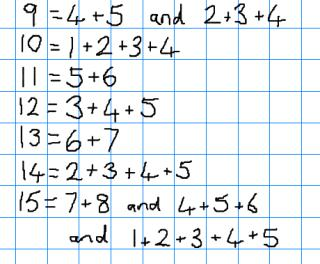You may also likeCounting Factors

Is there an efficient way to work out how many factors a large number has?Helen's Conjecture

Helen made the conjecture that "every multiple of six has more factors than the two numbers either side of it". Is this conjecture true?Oh! Hidden Inside?

Find the number which has 8 divisors, such that the product of the divisors is 331776.

Summing Consecutive Numbers

Age 11 to 14 Challenge Level:

Watch the video below to see how numbers can be expressed as sums of consecutive numbers.

Investigate the questions posed in the video and any other questions you come up with.

Can you draw any conclusions?
Can you support your conclusions with convincing arguments or proofs?

If you are unable to view the video, click below to reveal an alternative version of the problem.

Charlie has been thinking about sums of consecutive numbers. Here is part of his working out:Alison looked over Charlie's shoulder:

"I wonder if we could write every number as the sum of consecutive numbers?"

"Some numbers can be written in more than one way! I wonder which ones?"

"$9$, $12$ and $15$ can all be written using three consecutive numbers. I wonder if all multiples of $3$ can be written in this way?"

"Maybe you could write the multiples of $4$ if you used four consecutive numbers..."

Choose some of the questions above, or pose some questions of your own, and try to answer them.

Can you support your conclusions with convincing arguments or proofs?